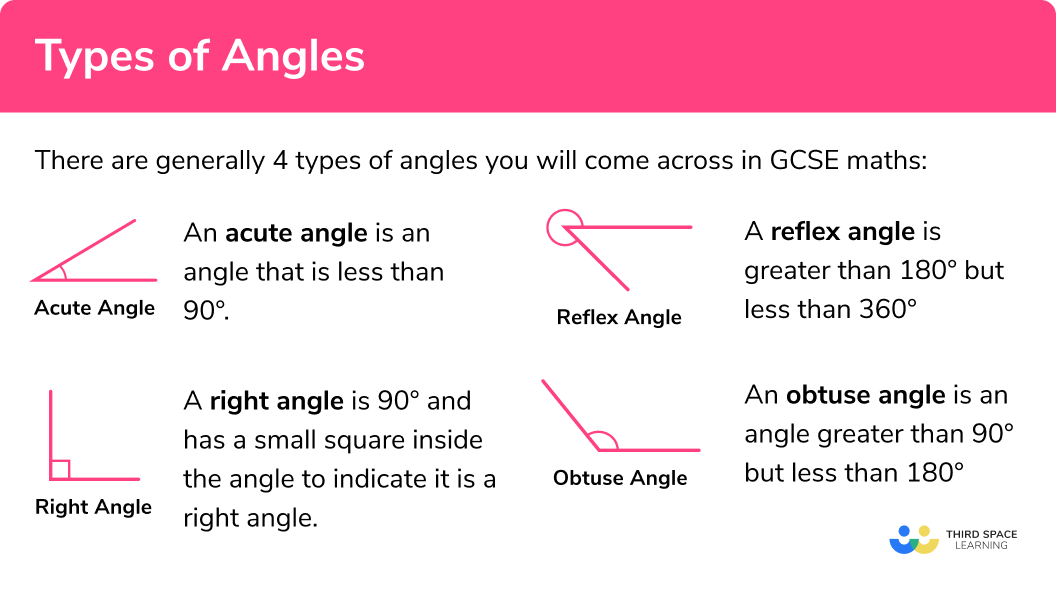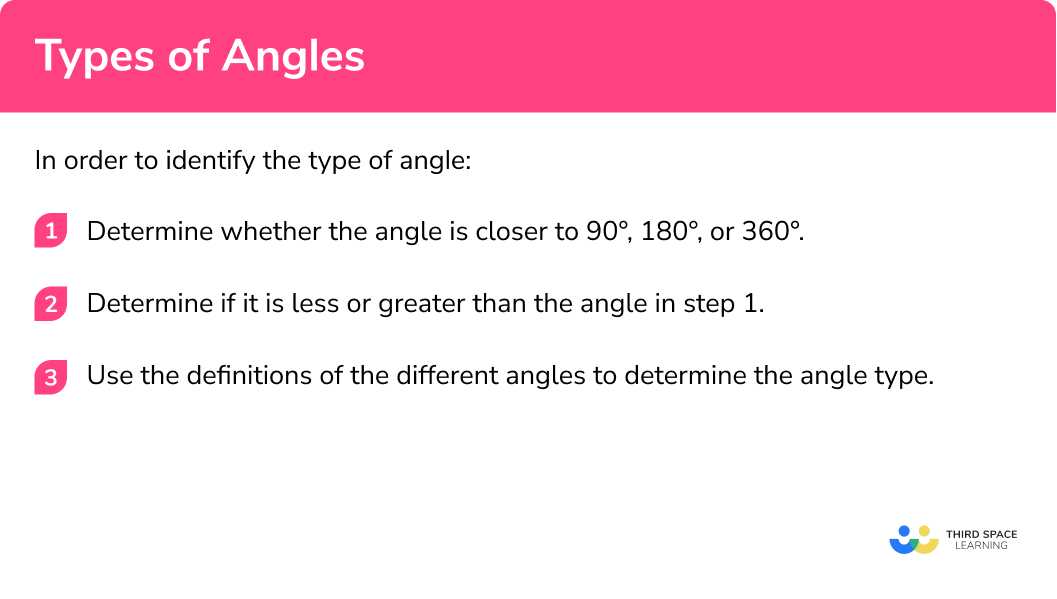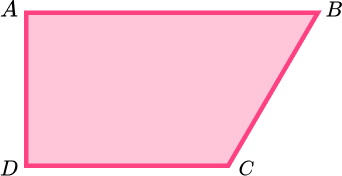# Types Of Angles

Here we will learn about the different types of angles including how to identify angle types and estimate them.

There are also types of angles worksheets based on Edexcel, AQA and OCR exam questions, along with further guidance on where to go next if you’re still stuck.

## What are the different types of angles?

An angle is formed between two lines (rays) meeting around a common point (vertex).

They are measured in degrees and have the degree sign °.

An angle is indicated by the symbol (∠) followed by three capital letter with the middle letter as the vertex of the angle.

The name of the angle on the left is ∠ABC or ∠CBA.

There are generally 4 types of angles you will come across in GCSE maths:

Acute Angles – An acute angle is an angle that is less than 90°:

Obtuse Angles – An obtuse angle is an angle greater than 90° but less than 180°:

Reflex Angles – A reflex angle is greater than 180° but less than 360°:

Right Angles – A right angle is 90° and has a small square inside the angle to indicate it is a right angle. The two rays in a right angle are perpendicular:

It is also important to note:

Straight Angle – A straight angle is one-half a whole turn and is 180°. It is the angle formed by the rays going in opposite directions.

Complete turn – A full rotation is 360°. Sometimes referred to as a full angle.

Did you know the word angle comes from the Latin word angulus, which means a corner?

### What are the different types of angles?## How to identify the type of angle

In order to find the angle type:

1. Determine whether the angle is closer to 90°, 180°, or 360°.
2. Determine if it is less or greater than the angle in step 1.
3. Use the definitions of the different angles to determine the angle type.

### How to identify the type of angle## Types of angles examples

### Example 1: classify angle given a diagram

What type of angle is seen below?

1. Determine whether the angle is closer to 90°, 180°, or 360°.

On examination the above angle is closest to 90°.

2Determine if it is less or greater than the angle in step 1.

Less than 90°.

3Use the definitions of the different angles to determine the angle type.

Since the angle is less than 90° it must be an acute angle.

### Example 2: classify angle given a measure

An angle is 290°. What type of angle is it?

The angle is closest to 360°.

290° is less than 360°.

Since the angle is less than 360° but greater than 180° it must be a reflex angle.

### Example 3: estimating the size of an angle

Estimate the size of the missing angle.

In the above example, the given angle is closer to the 90° line rather than the 180° line. Therefore a suitable estimate could be 120°.

### Common misconceptions

• Looking at the incorrect angle in the question

Particularly when it comes to reflex angles make sure to look at the angle indicated by the arc and not the acute angle.

• Forgetting the degree sign

When measuring or estimating angles your answer must always be given in degrees.

### Practice types of angles questions

1. State the name of the angle in the diagram below:Acute angleRight angleObtuse angleReflex angleThe mark in the corner tells us that this is 90° , so it is a right angle.

2. State the the name of the angle in the diagram below.Acute angleRight angleObtuse angleReflex angleThis angle is greater than 90° , and less than 180° , so it is an obtuse angle.

3. An angle is 87.8° . What type of angle is it?

Acute angleRight angleObtuse angleReflex angleThis angle is less than 90° , so it is an acute angle.

4) An angle is 298° . What type of angle is it?

Acute angleRight angleObtuse angleReflex angleThis angle is greater than 180° and less than 360° , so it is a reflex angle.

5. Which estimate best describes the size of the angle below:Approximately 30°Approximately 150°Approximately 220°Approximately 290°The angle is between 270° and 360° , so 290° is a reasonable estimate.

6. Which estimate best describes the size of the angle below:Approximately 120°Approximately 240°Approximately 60°Approximately 280°The angle is between 180° and 270° , so 240° is a reasonable estimate.

### Types of angles GCSE questions

1. (a) What is the special name for the angle below:(b) Estimate the size of the angle above.

(2 marks)

Acute angle

(1)

Accept any answer between  and 90°

(1)

2. Below is a trapezium.(a) Mark the acute angle with an X.

(b) Mark the obtuse angle with an O.

(c) Mark the right angle with an R.

(3 marks)

Marks ∠ABC with an X.

(1)

Marks ∠DCB  with an O.

(1)

Marks either ∠BAD or ∠CDA with an R.

(1)

3.

a) What is the mathematical name of the shape below?b) Estimate the size of the obtuse angles in the shape above.

(2 marks)

Parallelogram

(1)

Accept any answer between 90° and 180°.

(1)

## Learning checklist

You have now learned how to:

• Use standard convention for labelling angles
• Identify an angle type as obtuse, acute, reflex, right, straight or full turn
• Estimate the size of any angle

## Still stuck?

Prepare your KS4 students for maths GCSEs success with Third Space Learning. Weekly online one to one GCSE maths revision lessons delivered by expert maths tutors.

Find out more about our GCSE maths tuition programme.